# Central Science: Chapter 11 Flashcards

Set Details Share
created 3 years ago by Chemistry4Life
3,275 views
updated 3 years ago by Chemistry4Life
College: First year, College: Second year, College: Third year, College: Fourth year
Subjects:
chemistry
Page to share:
Embed this setcancel
COPY
code changes based on your size selection
Size:
X

1

Crystalline solids ________.
A) have their particles arranged randomly
B) have ordered structures
C) are usually very soft
D) exist only at high temperatures
E) exist only at very low temperatures

B

2

In liquids, the attractive intermolecular forces are ________.
A) very weak compared with kinetic energies of the molecules
B) strong enough to hold molecules relatively close together
C) strong enough to keep the molecules confined to vibrating about their fixed lattice points
D) not strong enough to keep molecules from moving past each other
E) strong enough to hold molecules relatively close together but not strong enough to keep molecules from moving past each other

E

3

As a gaseous element condenses, the atoms become ________ and they have ________ attraction for one another.
A) more separated, more
B) more separated, less
C) closer together, more
D) closer together, less
E) larger, greater

C

4

A gas is ________ and assumes ________ of its container, whereas a liquid is ________ and assumes ________ of its container.
A) compressible, the volume and shape, not compressible, the shape of a portion
B) compressible, the shape, not compressible, the volume and shape
C) compressible, the volume and shape, compressible, the volume
D) condensed, the volume and shape, condensed, the volume and shape
E) condensed, the shape, compressible, the volume and shape

A

5

Together, liquids and solids constitute ________ phases of matter.
A) the compressible
B) the fluid
C) the condensed
D) all of the
E) the disordered

C

6

A) They flow and are highly ordered.
B) They are highly ordered and not compressible.
C) They flow and are compressible.
D) They assume both the volume and the shape of their containers.
E) They flow and are not compressible.

E

7

The strongest interparticle attractions exist between particles of a ________, and the weakest interparticle attractions exist between particles of a ________.
A) solid, liquid
B) solid, gas
C) liquid, gas
D) liquid, solid
E) gas, solid

B

8

Which species has London dispersion forces as the only intermolecular force?
A) CH3CH2OH
B) Ar
C) NH3
D) HBr
E) H2O

B

9

Which molecule has hydrogen bonding as the predominant intermolecular force?
A) CH4
B) C6H6
C) CH3OH
D) CO2
E) C4H10

C

10

Which species has London dispersion forces as the only intermolecular force?
A) KBr
B) HI
C) CH3OH
D) CH3CH3
E) CH3F

D

11

When KBr dissolves in water, aqueous K+ and Br- ions result. The force of attraction that exists between K+ and H2O is called a(n) ________ interaction.
A) dipole-dipole
B) ion-dipole
C) ion-ion
D) London dispersion force
E) hydrogen bonding

B

12

________ are particularly polarizable.
A) Small nonpolar molecules
B) Small polar molecules
C) Large nonpolar molecules
D) Large polar molecules
E) Large molecules, regardless of their polarity,

E

13

The ease with which the charge distribution in a molecule can be distorted by an external electrical field is called the ________.
A) electronegativity
B) hydrogen bonding
C) polarizability
D) volatility
E) viscosity

C

14

The intermolecular force(s) responsible for the fact that CH4 has the lowest boiling point in the set CH4, CH3CH3, CH3CH2CH3, CH3CH2CH2CH3 is/are ________.
A) hydrogen bonding
B) London dispersion forces
C) mainly hydrogen bonding but also dipole-dipole interactions
D) dipole-dipole interactions
E) mainly London-dispersion forces but also dipole-dipole interactions

B

15

Elemental iodine (I2) is a solid at room temperature. What is the major attractive force that exists among different I2 molecules in the solid?
A) London dispersion forces
B) dipole-dipole interactions
C) ionic-dipole interactions
D) covalent-ionic interactions
E) dipole-dipole attractions

A

16

Hydrogen bonding is a special case of ________.
A) London-dispersion forces
B) ion-dipole attraction
C) dipole-dipole attractions
D) ion-ion interactions
E) none of the above

C

17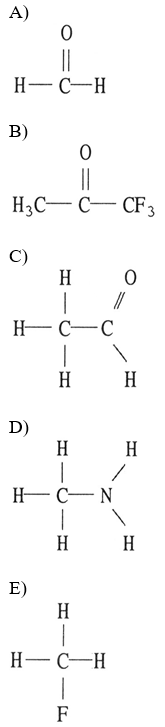Which one of the following substances will have hydrogen bonding as one of its intermolecular forces?

D

18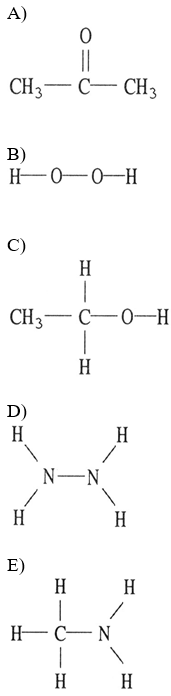Which one of the following substances will not have hydrogen bonding as one of its intermolecular forces?

A

19

What intermolecular force is responsible for the fact that ice is less dense than liquid water?
A) London dispersion forces
B) dipole-dipole forces
C) ion-dipole forces
D) hydrogen bonding
E) ionic bonding

D

20

The predominant intermolecular force in water is ________.
A) London dispersion forces
B) hydrogen bonding
C) ion-dipole forces
D) dipole-dipole forces
E) ionic bonding

B

21

Octane C8H18 molecules are held together by ________.
A) ion-ion interactions
B) hydrogen bonding
C) ion-dipole interactions
D) London dispersion forces
E) dipole-dipole interactions

D

22

Which of the following molecules has hydrogen bonding as its only intermolecular force?
A) HCl
B) NH3
C) H2O
D) CH3OH
E) None, all of the above exhibit dispersion forces.

E

23

Which of the following molecules has London Forces as its only intermolecular force?
A) H2O
B) CH3CH2NH2
C) HOCH2CH2OH
D) CH3CH3
E) None, all of the above exhibit dispersion forces.

D

24

What types of intermolecular forces exist between CH3OH and H2O?
A) dipole-dipole and ion-dipole
B) dispersion forces, dipole-dipole, and hydrogen bonding
C) dispersion forces, dipole-dipole, and ion-dipole
D) dispersion forces, hydrogen bonding, dipole-dipole, and ion-dipole
E) dispersion forces and ion-dipole

B

25

What type(s) of intermolecular forces exist between Cl2 and CCl4?
A) dispersion forces and ion-dipole
B) dispersion forces and dipole-dipole
C) dispersion forces
D) dispersion forces, ion-dipole, and dipole-dipole
E) None. Since both are gases at room temperature, they do not interact with each other.

C

26

What type(s) of intermolecular forces exist between NH3 and PO43-?
A) dispersion forces
B) dispersion forces, ion-dipole, and dipole-dipole
C) dispersion forces and dipole-dipole
D) dispersion forces and ion-dipole
E) dispersion forces, ion-dipole, dipole-dipole, and hydrogen bonds

D

27

What types of intermolecular forces exist between NH3 and H2O?
A) dispersion forces
B) dispersion forces and hydrogen bonds
C) dispersion forces and ion-dipole forces
D) dispersion forces, dipole-dipole forces, and hydrogen bonds
E) dispersion forces, hydrogen bonds, and ion-dipole forces

D

28

What types of intermolecular forces exist between water and HF?
A) dispersion forces and dipole-dipole forces
B) dispersion forces, dipole-dipole forces, and hydrogen bonds
C) dispersion forces and hydrogen bonds
D) dispersion forces
E) dispersion forces, hydrogen bonds, and ion-dipole forces

B

29

________ is the energy required to expand the surface area of a liquid by a unit amount of area.
A) Viscosity
B) Surface tension
C) Volatility
D) Meniscus
E) Capillary action

B

30

Which statements about viscosity are true?

(i) Viscosity increases as temperature decreases.
(ii) Viscosity increases as molecular weight increases.
(iii) Viscosity increases as intermolecular forces increase.

A) (i) only
B) (ii) and (iii)
C) (i) and (iii)
D) none
E) all

E

31

The shape of a liquid's meniscus is determined by ________.
A) the viscosity of the liquid
B) the type of material the container is made of
C) the relative magnitudes of cohesive forces in the liquid and adhesive forces between the liquid and its container
D) the amount of hydrogen bonding in the liquid
E) the volume of the liquid

C

32

Viscosity is ________.
A) the "skin" on a liquid surface caused by intermolecular attraction
B) the resistance to flow
C) the same as density
D) inversely proportional to molar mass
E) unaffected by temperature

B

33

How high a liquid will rise up a narrow tube as a result of capillary action depends on ________.
A) the magnitudes of cohesive forces in the liquid and adhesive forces between the liquid and the tube, and gravity
B) gravity alone
C) only the magnitude of adhesive forces between the liquid and the tube
D) the viscosity of the liquid
E) only the magnitude of cohesive forces in the liquid

A

34

The property responsible for the "beading up" of water is ________.
A) density
B) viscosity
C) vapor pressure
D) surface tension
E) hydrogen bonding

D

35

Heat of sublimation can be approximated by adding together ________ and ________.
A) heat of fusion, heat of condensation
B) heat of fusion, heat of vaporization
C) heat of freezing (solidification), heat of condensation
D) heat of freezing (solidification), heat of vaporization
E) heat of deposition, heat of vaporization

B

36

Which of the following statements is false?
A) The absolute value of the heat of sublimation is equal to the absolute value of the heat of deposition.
B) The heat of sublimation is equal to the sum of the heat of vaporization and the heat of deposition.
C) The heat of sublimation is equal to the sum of the heat of vaporization and the heat of freezing.
D) The absolute value of the heat of sublimation is equal to the absolute value of the sum of the heat of condensation and the heat of freezing.
E) The absolute value of the heat of deposition is equal to sum of the absolute value of the heat of vaporization and the absolute value of the heat of freezing.

C

37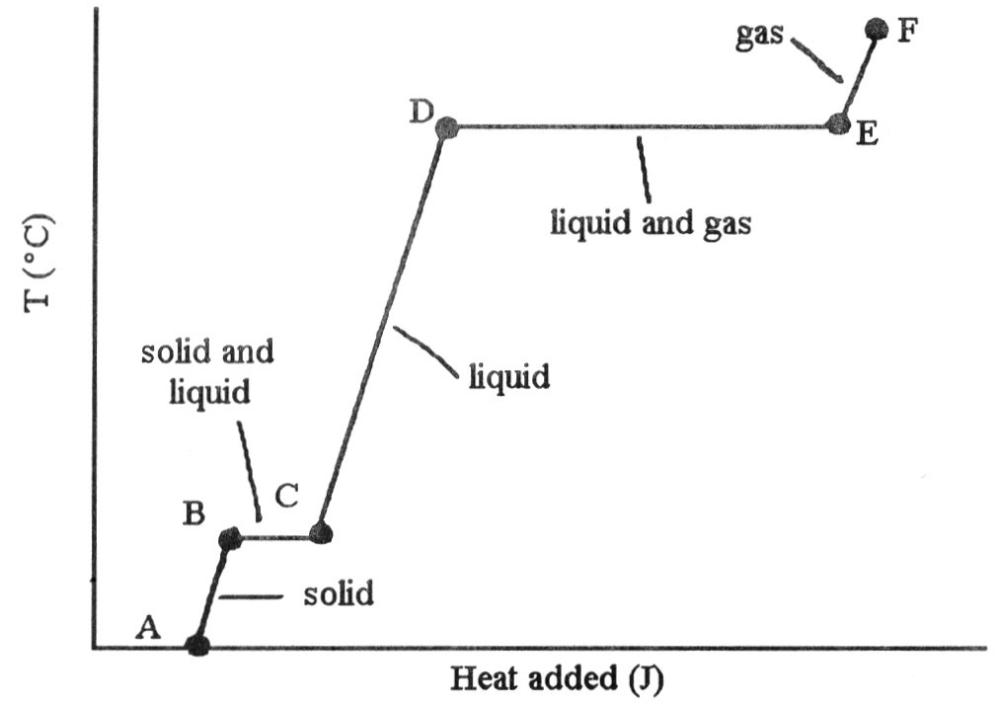The ________ (is)are associated with the heat energy being used up to increase distances between molecules.
A) phase change B → C
B) phase changes B → C and D → E
C) phase change D → E
D) phase change B → E
E) phase change C → E

B

38

Based on the following information, which compound has the strongest intermolecular forces?

Substance | ΔHvap (kJ/mol)
Argon (Ar) | 6.3
Benzene (C6H6) | 31.0
Ethanol (C2H5OH) | 39.3
Water (H2O) | 40.8
Methane (CH4) | 9.2

A) Argon
B) Benzene
C) Ethanol
D) Water
E) Methane

D

39

Which compound has the strongest intermolecular forces?
A) CCl4
B) CI4
C) CH4
D) H2
E) O2

B

40

Large intermolecular forces in a substance are manifested by ________.
A) low vapor pressure
B) high boiling point
C) high heats of fusion and vaporization
D) high critical temperatures and pressures
E) all of the above

E

41

A supercritical fluid can expand like a ________ to fill a container and has a density similar to that of a ________ so can behave as a solvent.
A) gas, plasma
B) gas, solid
C) solid, gas
D) liquid, gas
E) gas, liquid

E

42

The critical temperature and pressure of CS2 are 279 °C and 78 atm, respectively. A supercritical fluid can only exist at a temperature ________ 279 °C and pressure ________ 78 atm.
A) exactly, exactly
B) above, below
C) above, above
D) below, above
E) below, below

C

43

The substance with the largest heat of vaporization is ________.
A) H2
B) Cl2
C) I2
D) N2
E) O2

C

44

Of the following, ________ is an exothermic process.
A) melting
B) subliming
C) freezing
D) boiling
E) All of the above are exothermic.

C

45

Of the following, ________ should have the highest critical temperature.
A) CBr4
B) CCl4
C) CF4
D) CH4
E) H2

A

46

A volatile liquid is one that ________.
A) is highly flammable
B) is highly viscous
C) is highly hydrogen-bonded
D) is highly cohesive

E

47

In general, the vapor pressure of a substance increases as ________ increases.
A) surface tension
B) molecular weight
C) hydrogen bonding
D) viscosity
E) temperature

E

48

The vapor pressure of any substance at its normal boiling point is ________.
A) 1 Pa
B) 1 torr
C) 1 atm
D) equal to atmospheric pressure
E) equal to the vapor pressure of water

C

49

Volatility and vapor pressure are ________.
A) inversely proportional to one another
B) directly proportional to one another
C) not related
D) the same thing
E) both independent of temperature

B

50

Some things take longer to cook at high altitudes than at low altitudes because ________.
A) water boils at a lower temperature at high altitude than at low altitude
B) water boils at a higher temperature at high altitude than at low altitude
C) heat isn't conducted as well in low density air
D) natural gas flames don't burn as hot at high altitudes
E) there is a higher moisture content in the air at high altitude

A

51

The vapor pressure of a liquid ________.
A) increases linearly with increasing temperature
B) increases nonlinearly with increasing temperature
C) decreases linearly with increasing temperature
D) decreases nonlinearly with increasing temperature
E) is totally unrelated to its molecular structure

B

52

-ΔHvap/R is the slope of a plot of the natural log of the vapor pressure of a substance versus ________.
A) -1/T
B) -T
C) 1/T
D) T
E) 2T

C

53

On a phase diagram, the critical pressure is ________.
A) the pressure required to melt a solid
B) the pressure below which a substance is a solid at all temperatures
C) the pressure above which a substance is a liquid at all temperatures
D) the pressure at which a liquid changes to a gas
E) the pressure required to liquefy a gas at its critical temperature

E

54

On a phase diagram, the critical temperature is ________.
A) the temperature below which a gas cannot be liquefied
B) the temperature above which a gas cannot be liquefied
C) the temperature at which all three states are in equilibrium
D) the temperature required to melt a solid
E) the temperature required to cause sublimation of a solid

B

55

On a phase diagram, the melting point is the same as ________.
A) the triple point
B) the critical point
C) the freezing point
D) the boiling point
E) the vapor-pressure curve

C

56

When the phase diagram for a substance has a solid-liquid phase boundary line that has a ________ slope, the substance can go from solid to liquid, within a small temperature range, via the application of pressure.
A) positive
B) zero
C) y = 1
D) negative
E) y = 0

D

57

The predominant intramolecular force in CaBr2 is ________.
A) London-dispersion forces
B) ion-dipole forces
C) ionic bonding
D) dipole-dipole forces
E) hydrogen bonding

C

58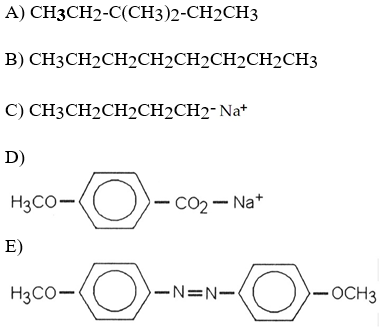Which of the following is most likely to exhibit liquid-crystalline behavior?

E

59

All of the following are characteristics of liquid crystal behavior except ________.
A) long axial structure
B) carbon-carbon single bonds
C) double bonding
D) ionic configuration
E) polar groups

D

60

In the ________ liquid-crystalline phase,the molecules are arranged in sheets, with their long axes parallel and their ends aligned as well.
A) smectic B
B) smectic C
C) smectic A
D) smectic E
E) smectic D

C

61

In the ________ liquid crystalline phase, the component molecules exhibit ________ dimensional ordering.
A) nematic, one
B) smectic A, one
C) nematic, two
D) nematic, three
E) smectic B, one

A

62

What are the common types of smectic liquid-crystalline phases?
A) A, C, and D
B) A only
C) A and C
D) A and D
E) C and D

C

63

________ liquid crystals are colored because the molecular layers are arranged in slightly twisted planes with respect to one another.
A) smectic B
B) cholesteric
C) smectic A
D) smectic C
E) smectic D

B

64

Molecules with ________ do not generally exhibit liquid-crystalline properties because they lack the rigidity necessary for alignment.
A) both single and double bonds
B) double or triple bonds
C) single, double, and triple bonds
D) only single bonds
E) only single and double bonds

D

65

For a given substance that exhibits liquid-crystalline properties, the transition from solid to liquid-crystal state occurs ________.
A) over a range of temperatures between the melting point of the solid and the boiling point of the liquid
B) at the melting point of the solid
C) over a range of temperatures that includes the melting point of the solid
D) at a well-defined temperature above the melting point of the solid
E) at a well-defined temperature below the melting point of the solid

B

66

There are ________ types of smectic liquid-crystalline phases.
A) 6
B) 5
C) 2
D) 3
E) 4

D

67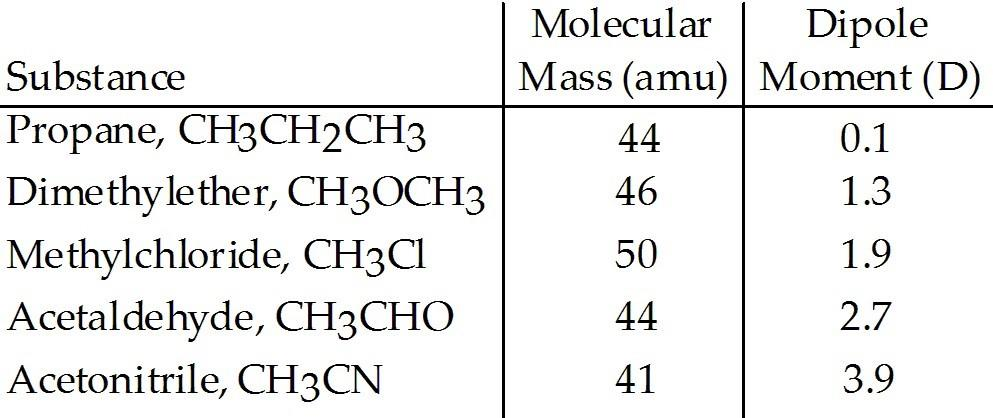Based on molecular mass and dipole moment of the five compounds in the table below, which should have the highest boiling point?

A) CH3CH2CH3
B) CH3OCH3
C) CH3Cl
D) CH3CHO
E) CH3CN

E

68The heating curve shown was generated by measuring the heat flow and temperature for a solid as it was heated. The slope of the ________ segment corresponds to the heat capacity of the liquid of the substance.
A) AB
B) BC
C) CD
D) DE
E) EF

C

69The heating curve shown was generated by measuring the heat flow and temperature for a solid as it was heated. The slope of the ________ segment corresponds to the heat capacity of the solid.
A) AB
B) BC
C) CD
D) DE
E) EF

A

70The heating curve shown was generated by measuring the heat flow and temperature for a solid as it was heated. The slope of the E-F segment corresponds to the heat capacity of the ________.
A) liquid-gas
B) solid-gas
C) gas
D) solid
E) liquid

C

71The heating curve shown was generated by measuring the heat flow and temperature of a solid as it was heated. The heat flow into the sample in the segment D-E will yield the value of the ________ of this substance.
A) ΔHsub
B) ΔHvap
C) ΔHmelting
D) ΔHfusion
E) ΔHrxn

B

72The heating curve shown was generated by measuring the heat flow and temperature of a solid as it was heated. The heat flow into the sample in the segment ________ will yield the value of the ΔHfusion of this substance.
A) AB
B) BC
C) CD
D) DE
E) EF

B

73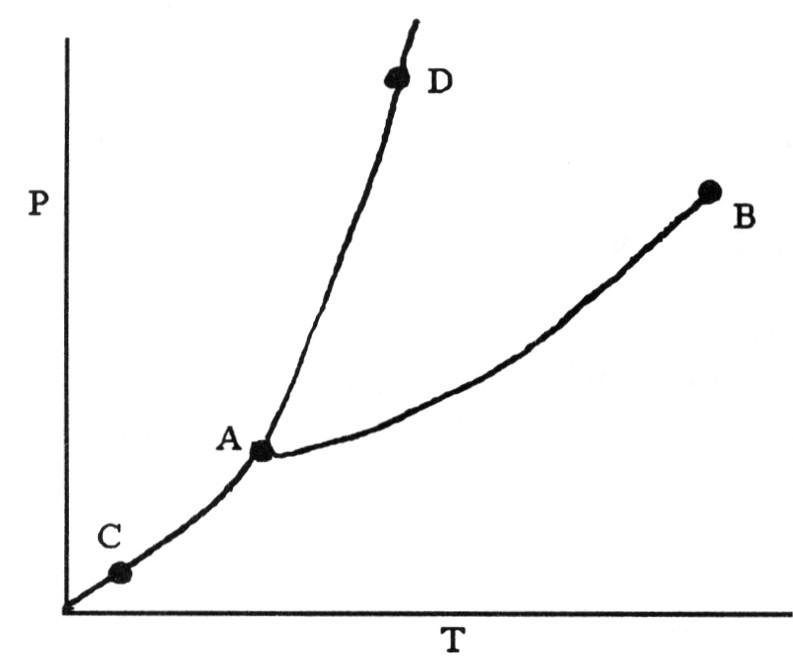On the phase diagram shown above, segment ________ corresponds to the conditions of temperature and pressure under which the solid and the gas of the substance are in equilibrium.
A) AB
B) AC
D) CD
E) BC

B

74On the phase diagram shown above, the coordinates of point B corresponds to the ________.
A) critical temperature and pressure
B) critical pressure
C) critical temperature
D) boiling point
E) triple point

A

75On the phase diagram shown above, the coordinates of point ________ correspond to the triple point.
A) A
B) B
C) C
D) D
E) E

A

76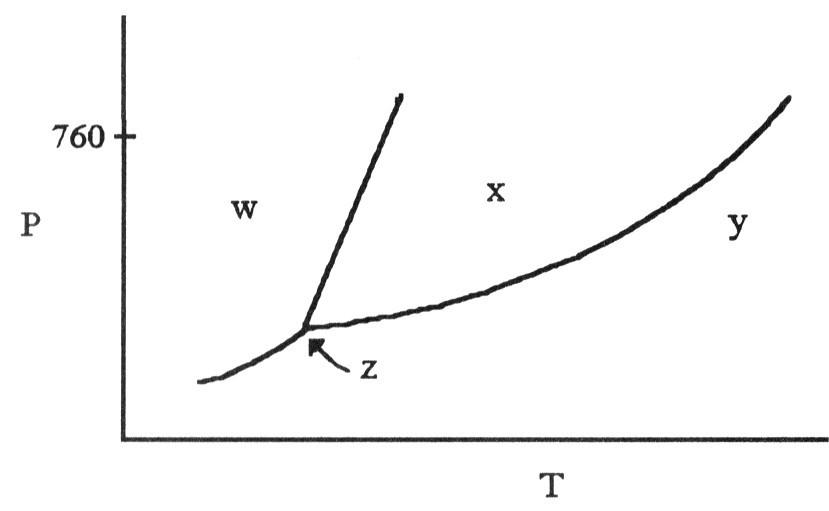The phase diagram of a substance is given above. The region that corresponds to the solid phase is ________.
A) w
B) x
C) y
D) z
E) x and y

A

77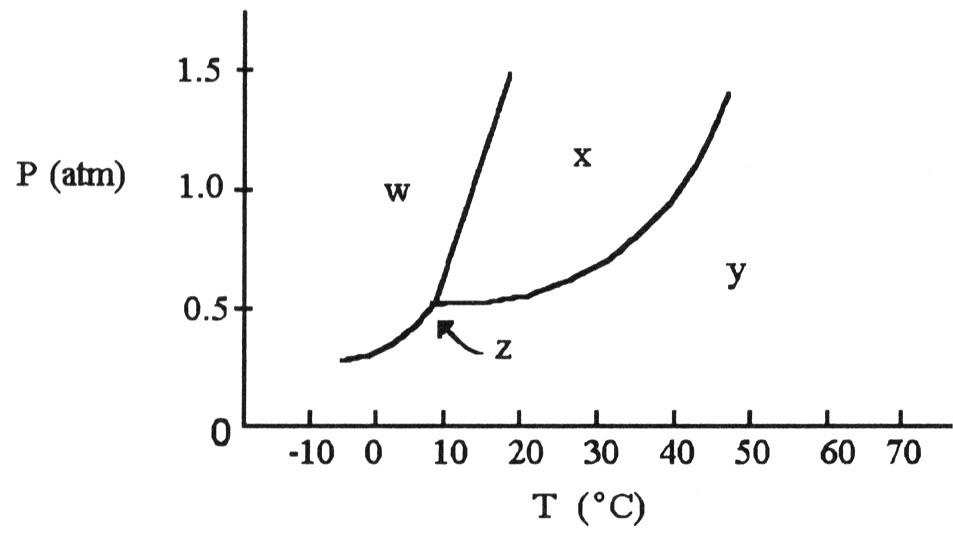According to the phase diagram shown above, what is the normal boiling point (°C)?
A) 10
B) 20
C) 30
D) 40
E) 50

D

78According to the phase diagram shown above, what is the normal melting point (°C)?
A) 15
B) 25
C) 35
D) 45
E) 55

A

79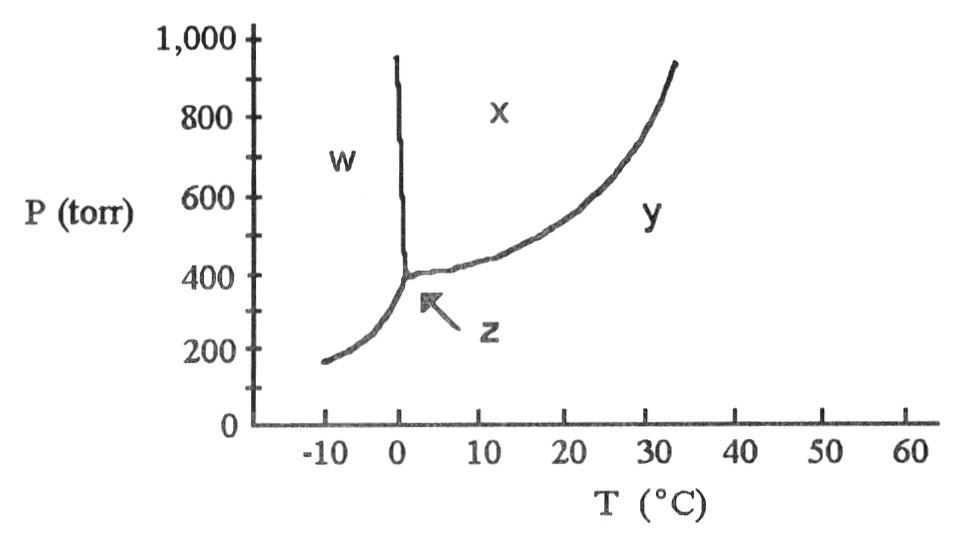The phase diagram of a substance is shown above. The area labeled ________ indicates the gas phase for the substance.
A) w
B) x
C) y
D) z
E) y and z

C

80The phase diagram of a substance is shown above. The area labeled ________ indicates the solid phase for the substance.
A) w
B) x
C) y
D) z
E) y and z

A

81The phase diagram of a substance is shown above. The area labeled ________ indicates the liquid phase for the substance.
A) w
B) x
C) y
D) z
E) y and z

B

82According to the phase diagram shown above, what is the normal boiling point (°C)?
A) 10
B) -3
C) 38
D) 29
E) 0

D

83According to the phase diagram shown above, what is the normal melting point (°C)?
A) -13
B) 0
C) 38
D) 10
E) 29

B

84

A ________ liquid crystal has the least order and is the most liquid-like.
A) nematic
B) smectic
C) cholesteric
D) smectic C
E) smectic B

A

85

________ liquid crystals are colored and change color with temperature changes.
A) Smectic A
B) Nematic
C) Cholesteric
D) Smectic B
E) Smectic C

C

86

As a gaseous element condenses, the atoms become ________ and they have ________ attraction for one another.
A) less separated, more
B) less separated, less
C) more separated, more
D) more separated, less
E) smaller, lesser

A

87

What is the predominant intramolecular force in NaNO3?
A) ionic bonding
B) ion-dipole attraction
C) dipole-dipole attraction
D) hydrogen bonding
E) London-dispersion forces

A

88

With what compound will NH3 experience only dispersion intermolecular forces?
A) BF3
B) LiF
C) CH3I
D) CH3OH
E) HCN

A

89

With what compound will NH3 experience only ion-dipole intermolecular forces?
A) LiCl
B) SiH4
C) CH3I
D) C3H7OH
E) OCl2

A

90

Which one of the following exhibits dipole-dipole attraction between molecules?
A) HCl
B) CF4
C) CS2
D) F2
E) BI3

A

91

Which one of the following exhibits dipole-dipole attraction between molecules?
A) NH3
B) CF4
C) C10H22
D) O2
E) SF6

A

92

Of the following substances, only ________ has London dispersion forces as its only intermolecular force.
A) CCl4
B) SnF3
C) CH3OH
D) HI
E) H2O

A

93

Which of the following has London dispersion forces as its only intermolecular force?
A) NBr3
B) CH3COOH
C) SiCl4
D) HBr
E) Cl2O

C

94

Which molecule has the lowest boiling point?
A) CH3CH2CH2CH3
B) CH3CH2CH3
C) CH3CH3
D) CH4
E) H2O

D

95

Which one of the following should have the lowest boiling point?
A) CH4
B) PCl3
C) C2H5COOH
D) LiCl
E) Cl2S

A

96

Of the following substances, ________ has the highest boiling point.
A) H2O
B) SiH4
C) Ar
D) Cl2
E) BF3

A

97

Of the following substances, ________ has the highest boiling point.
A) HOCH2CH2CH2OH
B) CH3CH2OH
C) C4H10
D) N2
E) Cl2

A

98

Of the following substances, ________ has the highest boiling point.
A) Br2
B) N2
C) Cl2
D) O2
E) H2

A

99

Which one of the following derivatives of methane has the highest boiling point?
A) CI4
B) CBr4
C) CCl4
D) CF4
E) CH4

A

100

What is the predominant intermolecular force in CH4?
A) London-dispersion forces
B) ion-dipole attraction
C) ionic bonding
D) dipole-dipole attraction
E) hydrogen bonding

A

101

What is the predominant intermolecular force in H2NNH2?
A) hydrogen bonding
B) ion-dipole attraction
C) ionic bonding
D) dipole-dipole attraction
E) London-dispersion forces

A

102

What is the predominant intermolecular force in HCN?
A) dipole-dipole attraction
B) ion-dipole attraction
C) ionic bonding
D) hydrogen bonding
E) London dispersion forces

A

103

Ethanol melts at -114 °C and boils at 78 °C at a constant pressure of 1 atm. What state of matter must a sample of ethanol be in at 0°C and 1 atm?
A) liquid
B) gas
C) solid
D) solid and liquid in equilibrium
E) liquid and gas in equilibrium

A

104

At 1 atm, an unknown sample melts at 49.9 °C and boils at 209.5 °C. If the temperature is 0°C, what is the state of matter for the sample?
A) solid and liquid in equilibrium
B) liquid
C) gas
D) solid
E) liquid and gas in equilibrium

D

105

The enthalpy change for converting 1.00 mol of ice at -25.0 °C to water at 50.0 °C is ________ kJ. The specific heats of ice, water, and steam are 2.09 J/g-K, 4.18 J/g-K, and 1.84 J/g-K, respectively. For H2O, ΔHfus = 6.01 kJ/mol, and ΔHvap = 40.67 kJ/mol.
A) 12.28
B) 6.27
C) 10.71
D) 4709
E) 8.83

C

106

The enthalpy change for converting 10.0 g of ice at -50.0 °C to water at 50.0 °C is ________ kJ. The specific heats of ice, water, and steam are 2.09 J/g-K, 4.18 J/g-K, and 1.84 J/g-K, respectively. For H2O, ΔHfus = 6.01 kJ/mol, and ΔHvap = 40.67 kJ/mol.
A) 12.28
B) 4.38
C) 3138
D) 6.47
E) 9.15

D

107

The heat of fusion of water is 6.01 kJ/mol. The heat capacity of liquid water is 75.3 J/mol ∙ K. The conversion of 50.0g of ice at 0.00 °C to liquid water at 0.00°C requires ________ kJ of heat.
A) 6.01
B) 16.7
C) 75.3
D) 17.2
E) Insufficient data are given.

B

108

Calculate the enthalpy change (in kJ) associated with the conversion of 25.0 grams of ice at -4.00 °C to water vapor at 109.0 °C. The specific heats of ice, water, and steam are 2.09 J/g-K, 4.18 J/g-K, and 1.84 J/g-K, respectively. For H2O, ΔHfus = 6.01 kJ/mol, and ΔHvap = 40.67 kJ/mol.
A) 64.8
B) 75.9
C) 11100
D) 12000
E) 112

B

109

Ethanol ( OH) melts at -114 °C. The enthalpy of fusion is 5.02 kJ/mol. The specific heats of solid and liquid ethanol are 0.97 J/g-K and 2.3 J/g-K, respectively. How much heat (kJ) is needed to convert 25.0 g of solid ethanol at -135 °C to liquid ethanol at -60 °C?
A) 207.3
B) -13.3
C) 6.34
D) 3617
E) 8.63

C

110

Of the following, ________ is the most volatile.
A) C2H6
B) C2I6
C) C2Br6
D) C2Cl6
E) C2F6

A

111

Which molecule is the least volatile?
A) CH3Cl
B) CH3I
C) CH3F
D) CH4
E) CH3Br

B

112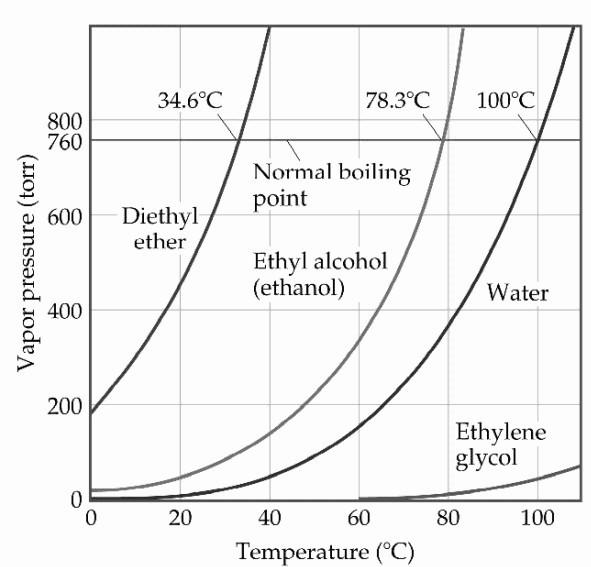Based on the figure above, the boiling point of diethyl ether under an external pressure of 0.605atm is ________ °C.
A) 40
B) 10
C) 30
D) 20
E) 0

D

113Based on the figure above, the boiling point of diethyl ether under an external pressure of 0.658atm is ________ °C.
A) 20
B) 40
C) 60
D) 80
E) 70

E

114

Based on the figure above, what is the boiling point (°C) of water under an external pressure of 0.724atm.
A) 40
B) 60
C) 70
D) 90
E) 80

D

115The phase diagram of a substance is given above. This substance is a ________ at 30 °C and 0.5 atm.
A) liquid
B) gas
C) solid
D) supercritical fluid
E) crystal

B

116

In general, intramolecular forces determine the ________ properties of a substance and intermolecular forces determine its ________ properties.

chemical, physical

117

London Dispersion Forces tend to ________ in strength with increasing molecular weight.

increase

118

The conversion of a solid to a liquid is called ________.

melting

119

The initial discovery of a liquid crystal resulted from studies of a ________ derivative.

cholesterol

120

The principal source of the difference in the normal boiling points of ICl (97 °C; molecular mass 162 amu) and Br2 (59 °C; molecular mass 160 amu) is both dipole-dipole interactions and London dispersion forces.

false

121

The boiling points of normal hydrocarbons are higher than those of branched hydrocarbons of similar molecular weight because the London-dispersion forces between normal hydrocarbons are greater than those between branched hydrocarbons.

true

122

Heats of vaporization are greater than heats of fusion.

true

123

Under ordinary conditions, a substance will sublime rather than melt if its triple point occurs at a pressure above atmospheric pressure.

true

124

Molecules containing many double bonds do not exhibit liquid-crystal behavior because free rotation can occur only around single bonds making these molecules rigid.

false